# Getting started with a simple example

library(rhosa)

This is a simple example, based on the outline at Figure 1 of , which demonstrates how to use rhosa’s functions to find an obscure relationship between two frequencies in some time series imitated by a generative model.

With four cosinusoidal waves having arbitrarily different phases (omega_a, omega_b, omega_c, and omega_d), but sharing a couple of frequencies (f_1 and f_2), we define function D(t) to simulate a pair of time series: v and w. We make them noisy by adding an independent random variate that follows the standard normal distribution.

set.seed(1)
f_1 <- 0.35
f_2 <- 0.2
D <- function(t) {
omega_a <- runif(1, min = 0, max = 2 * pi)
omega_b <- runif(1, min = 0, max = 2 * pi)
omega_c <- runif(1, min = 0, max = 2 * pi)
omega_d <- runif(1, min = 0, max = 2 * pi)
wave_a <- function(t) cos(2 * pi * f_1 * t + omega_a)
wave_b <- function(t) cos(2 * pi * f_2 * t + omega_b)
wave_c <- function(t) cos(2 * pi * f_1 * t + omega_c)
wave_d <- function(t) cos(2 * pi * f_2 * t + omega_d)
curve_v <- function(t) wave_a(t) + wave_b(t) + wave_a(t) * wave_b(t)
curve_w <- function(t) wave_c(t) + wave_d(t) + wave_c(t) * wave_b(t)
data.frame(v = curve_v(t) + rnorm(length(t)),
w = curve_w(t) + rnorm(length(t)))
}

Both v and w are oscillatory in principle:

data <- D(seq_len(2048))
with(data, {
plot(seq_len(100), head(v, 100), type = "l", col = "green", ylim = c(-3, 3), xlab = "t", ylab = "value")
lines(seq_len(100), head(w, 100), col = "orange")
})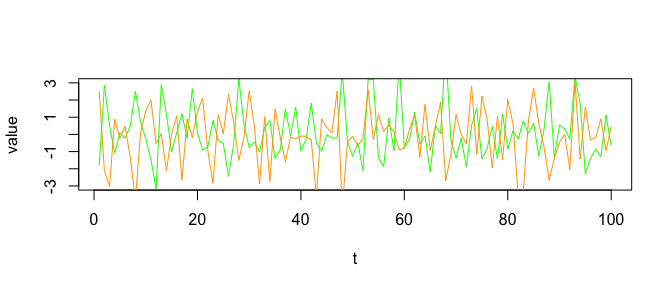v and w.

It is noteworthy that the power spectrum densities of v and w are basically identical as shown in their spectral density estimation:

with(data, {
spectrum(v, main = "v", col = "green")
spectrum(w, main = "w", col = "orange")
})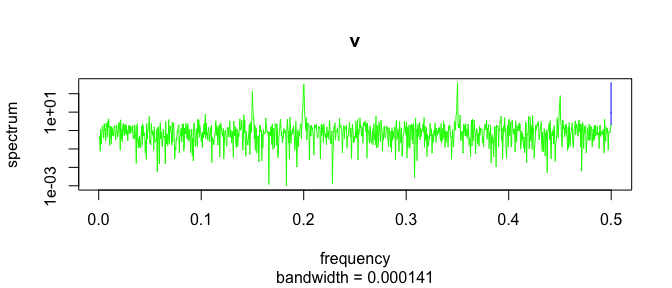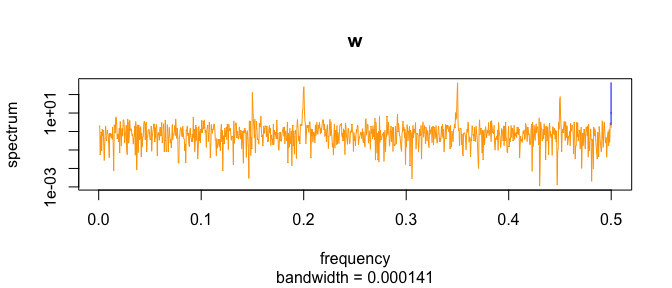On the other hand, their bispectra are different. More specifically, we are going to see that their bicoherence at some pairs of frequencies are different. rhosa’s bicoherence function allows us to estimate the magnitude-squared bicoherence from samples.

x <- replicate(100, D(seq_len(128)), simplify = FALSE)
m_v <- do.call(cbind, Map(function(d) {d$v}, x)) m_w <- do.call(cbind, Map(function(d) {d$w}, x))

library(rhosa)

bc_v <- bicoherence(m_v, window_function = 'hamming')
bc_w <- bicoherence(m_w, window_function = 'hamming')

In the above code, we take 100 samples of the same length for a smoother result. The bicoherence function accepts a matrix whose column represents a sample sequence, and returns a data frame. Note that an optional argument to bicoherence is given for requesting tapering with Hamming window function.

library(ggplot2)

plot_bicoherence <- function(bc) {
ggplot(bc, aes(f1, f2)) +
geom_raster(aes(fill = value)) +
scale_fill_gradient(limits = c(0, 10)) +
coord_fixed()
}

The axis f1 and f2 represent normalized frequencies in unit cycles/sample of range [0, 1). Frequency pairs of bright points in the following plot of bc_v indicate the existence of some quadratic phase coupling, as expected:

plot_bicoherence(bc_v)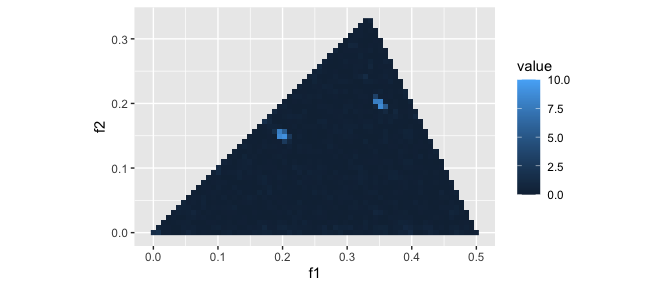v’s estimated magnitude-squared bicoherence.

In contrast, bc_w has no peaks at frequency pair (f_1, f_2) = (0.35, 0.2), etc.:

plot_bicoherence(bc_w)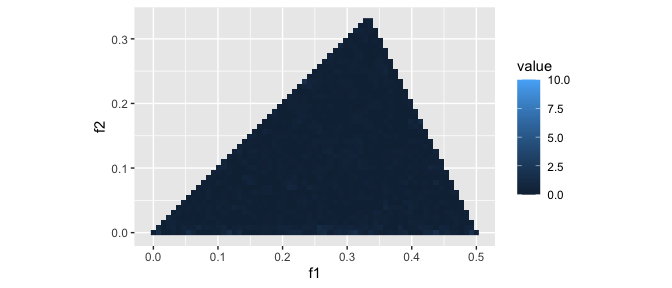w’s estimated magnitude-squared bicoherence.